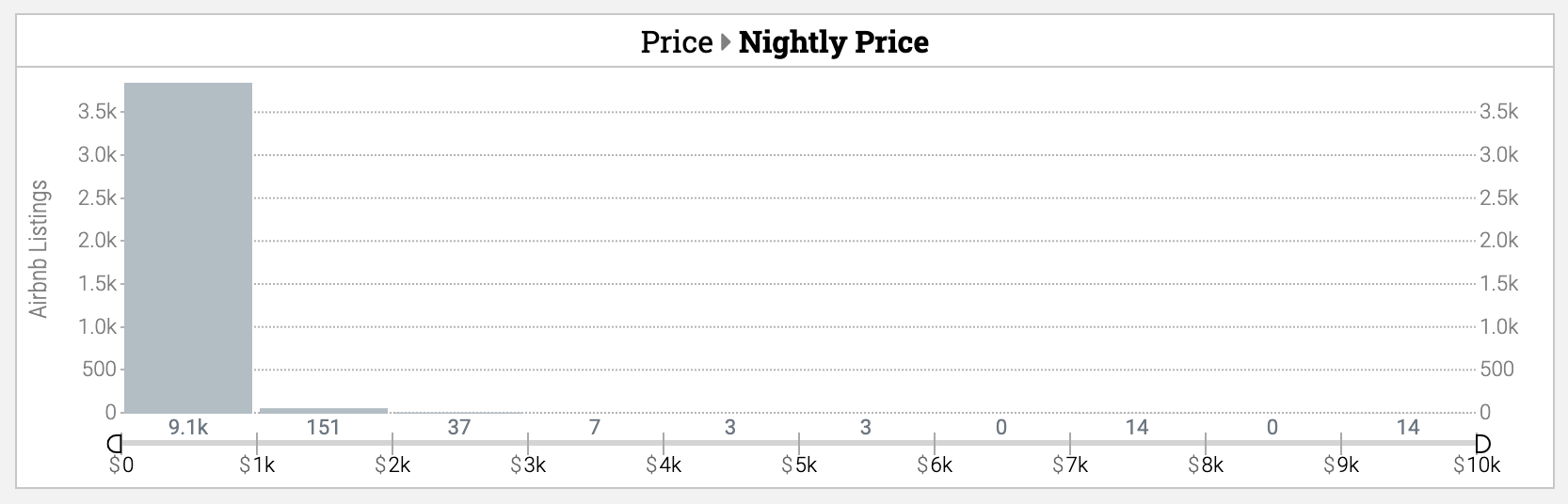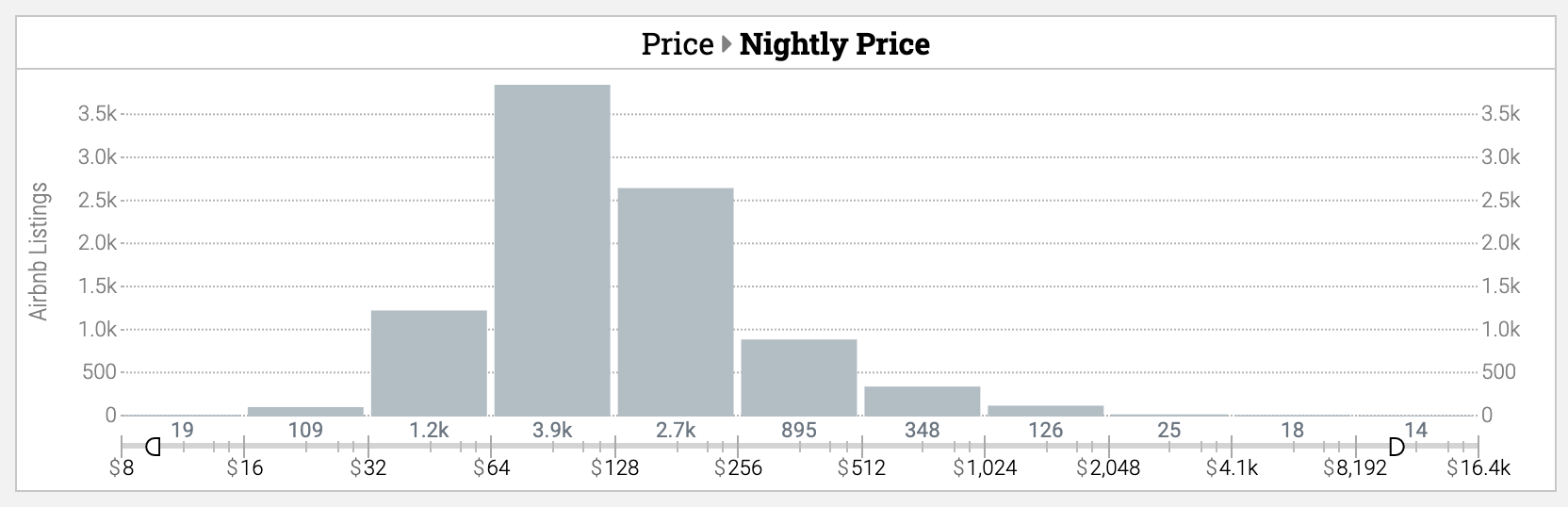# ◆ About Linear & Logarithmic Axis Scale Types

The axis scale is a key factor in revealing relevant trends in visual analytics, and selecting the appropriate type in an analysis setting can produce more informative and effective charts.

The type of axis defines the main mathematical model that transforms values or measurements to visual features of a chart, such as length of bars, position of lines or points, or colors of map regions or points.

Specifically, using a logarithmic scale can be an effective and balanced way to display your data when it spreads over a wide range of measurements or values.

#### Using a linear scale

• The linear scale is the more common, and straightforward, scale type.
• In a chart axis with a linear scale, the ticks (sample text values on the axis) follow a regular pattern with constant change, such as 2, 4, 6, 8, 10 and so on.
• With a logarithmic scale, adding a constant amount to the data results in a constant change of the visualized output.
• For example, changing data from 30 to 40 has the same effect in output (position, color, and so on) as going from 20 to 30, or 40 to 50.

#### Using a logarithmic (log) scale

• In a chart axis with a logarithmic scale, the ticks (sample text values on the axis) are less regular, and more exponential, with values such as, 4, 8, 16, 32 and so on.
• Even though each tick is evenly spaced, note that the values increase much faster in a log scale.
• With a logarithmic scale, the chance in output is not constant.
• For example, going from 10-to-100, and from 100-to-1000, can generate the same difference in output, such as the same length change of a bar in a bar chart. Thus, this scale would emphasize changes in smaller values, and de-emphasize changes in larger values.
• Logarithmic scale can be created using multiples of 2 (2,4,8,16,23...), or multiples of 10 (1,10,100,1000...).
• Keshif uses multiples of 10 in most settings, and multiples of 2 for binning. Learn more below.

### Differences between data value scales and measurement scales

Keshif visualizes two types of trends with different analytical powers and corresponding axis scale options.

#### Visualizing record data directly (for value axis)

A common type of direct visualization of record data is used in record charts.

Another direct use of record data is for the binning scale for aggregating numeric data (such as people by age groups) in histograms.

• In this setting, the logarithmic scale uses multiples of two. This helps to generate more optimum and evenly spaced intervals when data values are not too large.

#### Visualizing aggregate measurements (for measurement axis)

Visualizing measurements of groups of records ( average age of a group of people, or their count), in aggregate charts, such as categorical bar charts (such as gender), histograms (measuring average profit of items in a price range), or aggregated line charts based on timestamp data.

### Special Features

• Keshif uses a consistent approach for values axis scales of a data attribute across different visualization types, such as time-series value axis, scatter plot x/y axes, record map colors, and also histogram bins. Furthermore, for time-series data, this setting is also shared by the specific time-points. For example, if GDP data is best shown using logarithmic scale because of vast differences over time and countries, the histogram of GDPs in a specific year also uses logarithmic scale automatically.
• Keshif automatically checks data distribution trends, and applies linear or log scale to better fit underlying detected data characteristics.

### Constraints

• Logarithmic scale is not available when the visualized data (of numeric attributes or group measurements) includes zero or negative values.
• There can be additional constraints depending on chart and analytics type. For example, stacked charts cannot be used with logarithmic scales.

### Examples

In the charts below, most of the nightly prices are under \$1,000, but there are a handful of much larger values that skew the chart in linear scale. In contrast, comparing the nightly prices in logarithmic scale reduces that variation to make the chart easier to understand.

#### Binning with Linear Scale#### Binning with Log Scale